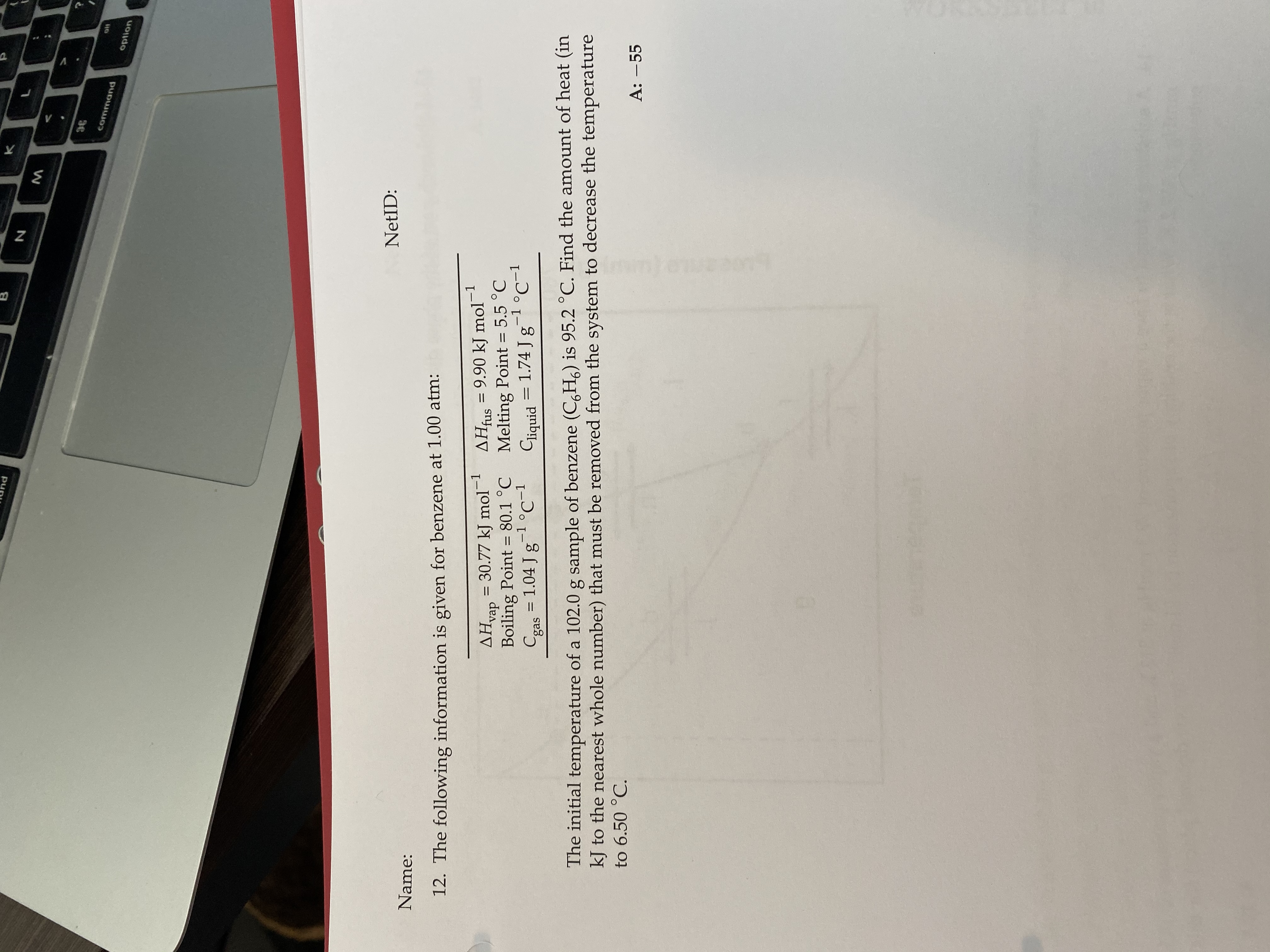# The following information is given for benzene at 1.00 atm: ΔHvap=30.77 kJ mol^-1 ΔHfus=9.90 kJ mol^-1 bp=80.1 degrees C mp=5.5 degrees C Cgas=1.04 J g^-1 degrees C^-1 Cliquid=1.74 J g^-1 degrees C^-1 The initial temperature of a 102.0 g sample of benzene (C6H6) is 95.2 degrees C. Find the amount of heat (in kJ to the nearest whole number) that must be removed from the system to decrease the temperature to 6.50 degrees C.